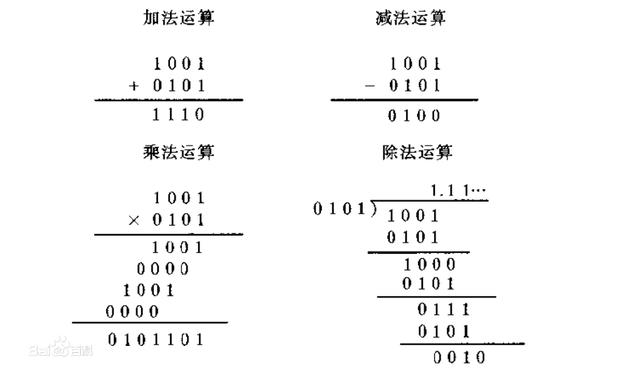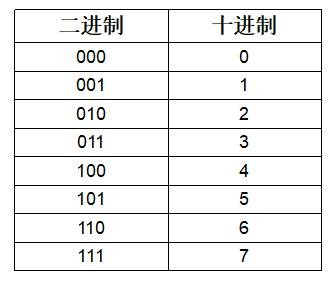• ## 二进制乘法原理

万次阅读 2015-03-21 21:29:41
二进制乘法原理：就是左移（进位）8次，每次最高位为1则加进去，8位移完就得出乘积了实际上和我们做10进制的乘法是一样的，只不过这里的进制是2罢了比如5×6，转成二进制就是0101×0110 十进制乘法大家都会做，公式...
二进制乘法原理：
1111B*1111B=11100001
1111
11110
111100
+1111000     二进制相加
------------------------
11100001

就是左移（进位）8次，每次最高位为1则加进去，8位移完就得出乘积了实际上和我们做10进制的乘法是一样的，只不过这里的进制是2罢了。
比如5×6，转成二进制就是0101×0110 十进制乘法大家都会做，公式就是我们他当成十进制101×110来计算下看看
4位乘积＝被乘数×千位被＋被乘数×百位＋被乘数×十位＋被乘数×个位既0101×0110＝101×0000＋101×100＋101×10＋101×0 变化下：
4位乘积＝被乘数×千位数×1000＋被乘数×百位数×100＋被乘数×10位数×10＋被乘数×个位数
既0101×0110＝101×（0×1000）＋101×（1×100） ＋101×（1×10）＋101×0
再变化下： 4位乘积＝被乘数×千位数×10×10×10＋被乘数×百位数×10×10＋被乘数×10位数×10＋被乘数×个位数
既0101×0110＝101×（0×10×10×10）＋101×（1×10×10）＋101×（1×10）＋101×0 ＝（（（101×0）×10）＋（101×1））×10＋（101×1））×10＋101×0
，实际上乘法结果就是被乘数乘以每一位乘以模（10）的N次方的累计和（其实左移位就是进位啦，看得出来吗？）而换成2进制的话很简单，把10读成二进制2就行了，结果还是：
4位乘积＝被乘数×千位数×10×10×10＋被乘数×百位数×10×10＋被乘数×10位数×10＋被乘数×个位数
既0101×0110＝101×（0×10×10×10）＋101×（1×10×10）＋101×（1×10）＋101×0 ＝（（（101×0）×2）＋（101×1））×2＋（101×1））×2＋101×0
由于乘2就是移位（进位），把上面的公式中乘2换成左移位就行了 PS：由于二进制只有0和1，乘2可以用左移一位来实现，也可以“自己加自己”来实现的，很多CPU的左移指令和“自己加自己”一样用软件乘法要耗费很多CPU时间，只要CPU有硬件乘法器，当然是用硬件的啦，哪会快很多的.

展开全文• 撰文：茂喵喵审核：伯毅在数学学习中，我们比较常用的是十进制，这在生活中也是一样。所谓十进制，就是在进行数字运算时，低位上的数字运算满十向上进位，或者低位上的数字不足时向高一位的...因此，二进制中，只...
撰文：茂喵喵审核：伯毅在数学学习中，我们比较常用的是十进制，这在生活中也是一样。所谓十进制，就是在进行数字运算时，低位上的数字运算满十向上进位，或者低位上的数字不足时向高一位的数字借位，满十是基础。这种进制，满足了我们的日常生活所需。但是，现代生活的必需品——电子产品，却是基于另一种进制的。这就是我们今天要讲到的——二进制。十进制是满十进一，二进制就是满二进一，这个规则是统一的。因此，二进制中，只有0和1这两个数。那么二进制究竟是如何运算的？我们具体来看看。加法：0+0=0，0+1=1，1+0=1，1+1=0；乘法：0×0=0，0×1=0，1×0=0，1×1=1；减法：0-0=0，1-0=1，0-1=1，1-1=0；除法：0÷1=0，1÷1=1；竖式计算如下：那么，计算机为什么要用二进制呢？1、二进制方便硬件实现。因为二进制只有0和1这两个数，而在我们日常生活中，经常遇到的状态也是有两种，比如：开关的开与闭，答案的对与错，电路的正常与不正常等，只有两种状态；2、二进制更适合复杂的计算机逻辑运算。因为在逻辑里面只有真与假两种状态，一般真为1，假为0；3、二进制运算更简单，可以减少很多因为运算复杂而带来的失误；4、二进制应用于通信，更加稳定可靠。十进制与二进制间的相互转化，我们列举一些常用的：日常我们所使用的电脑或手机，它们只读得懂0和1所组成的数组，也就是说，我们通过屏幕所看到的无论是文字还是视频、图片，在电脑的CPU中，只是一串串0、1字符，然后由此引发出现代编程语言，诸如早期的汇编语言，C语言，Java等，人类需要基础的语言，作为日常交流，自然机器也是需要的。当然这里，还要说一下我们日常所提到的内存空间。比如，一个U盘的存储空间为8G，也就是8Gb字节，这里的字节，就是二进制数据的单位，通常由8位0、1数字组成。通常情况下，一个汉字由占用字节，那么8Gb能存储多少汉字呢？8Gb=8×1024Mb=8×1024×1024×1024÷2B=40亿，也就是可以存储40亿个汉字，这里的B就表示字节。好了，今天的科普分享就到这里。下期预告：日常生活中有哪些我们不知道却在做的不环保行为
展开全文• 二进制的异或FWT实际上在做这样一件事： 构造一个行列式不为0的Len阶方阵T，使得TA⋅TB=TCTA\cdot TB=TCTA⋅TB=TC 将矩阵乘法拆开看，这个矩阵满足：∀x,i,j    T(x,i)T(x,j)=T(x,i⊕j)\forall ...
并不是什么新方法，老早就有了。
背景
二进制的异或FWT实际上在做这样一件事：
构造一个行列式不为0的Len阶方阵T，使得$TA\cdot TB=TC$
将矩阵乘法拆开看，这个矩阵满足：$\forall {x,i,j}~~~~T(x,i)T(x,j)=T(x,i\oplus j)$
只要单个位的数满足上述性质，我们不妨将T(x,y)构造成其每一位的T的乘积，显然也满足性质。
于是只需要找出一个2*2的矩阵便可以了。
对于二进制异或，这个矩阵可以是$[[1,1],[1,-1]]$，也可以是$[[1,-1],[1,1]]$
原理
K进制下对应的矩阵或许有很多，但是我们可以利用范德蒙德矩阵快速构造出一个
$\begin{bmatrix} 1& 1 & 1& ... & 1\\ 1& w_k^1& w_k^2& ... & w_k^{k - 1}\\ 1& w_k^2 & w_k^4& ... & w_k^{2(k - 1)}\\ ...& ...& ...& ...& ...\\ 1& w_k^{k - 1}& w_k^{2(k - 1)} & ... & w_k^{(k - 1)(k - 1)} \end{bmatrix}$
其中$w_k$是K次单位根。由于是范德蒙德矩阵，因此是有逆的。
$\frac{1}{k} \begin{bmatrix} 1& 1 & 1& ... & 1\\ 1& w_k^{-1}& w_k^{-2}& ... & w_k^{-(k - 1)}\\ 1& w_k^{-2} & w_k^{-4}& ... & w_k^{-2(k - 1)}\\ ...& ...& ...& ...& ...\\ 1& w_k^{-(k - 1)}& w_k^{-2(k - 1)} & ... & w_k^{-(k - 1)(k - 1)} \end{bmatrix}$
其实就是单位根取倒数，然后除去K.
朴素算法
有了上述矩阵及其逆矩阵之后，我们可以暴力计算$TA$,$TB$来得到$TC$，再得到C.
时间复杂度$O(n^2k)$，与暴力无异。
类FWT加速
我们将原数一位位的变化成贡献到的位置，并将系数逐步乘上。
具体地，做完低i位后，设x=a+b,b是低i位，那么a[x]的值就是所有原高位与a相同的位置对所有低位与b相同的位置的贡献之和。转移就是变化原数一位，乘上相应系数，加到对应位置下一次迭代。
也可以理解为分治，推式子。
时间复杂度$O(nKlog_Kn)$
#include <bits/stdc++.h>
using namespace std;
typedef long long ll;
const int mo = 1e9 + 9, K = 11, G = 13, N = 1e5;
int n, k;
ll T[K][K], NT[K][K];
ll a[N], b[N], w1, c[N], ans[N];
ll ksm(ll x, ll y) {
ll ret = 1; for (; y; y>>=1) {
if (y & 1) ret = ret * x % mo;
x = x * x % mo;
}
return ret;
}

int wi;
void fast_trans(ll T[K][K], ll *a) {
static ll b[N];
for(int m = 1; m < n; m *= k) {
memset(b, 0, sizeof b);
for(int i = 0; i < n; i += m * k) {
for(int j = 0; j < m; j++) {
for(int u = 0, wu = 0; u < k; u++, wu += m) {
for(int v = 0, wv = 0; v < k; v++, wv += m) {
b[i + j + wu] = (b[i + j + wu] + a[i + j + wv] * T[u][v]) % mo;
}
}
}
}
memcpy(a, b, sizeof b);
}
}

void trans(ll T[K][K], ll *a) {
fast_trans(T, a);
return;

static ll res[N];
memset(res, 0, sizeof res);
for(int i = 0; i < n; i++) {
for(int j = 0; j < n; j++) {
ll z = 1, ti = i, tj = j;
for(int w = 0; w < wi; w++) {
z = z * T[ti % k][tj % k] % mo;
ti /= k, tj /= k;
}
res[i] = (res[i] + z * a[j]) % mo;
}
}
memcpy(a, res, sizeof res);
}

int main() {
freopen("a.in","r",stdin);
freopen("b.out","w",stdout);
cin>>n>>k;
int y = n;
while (y) {
wi++, y/=k;
}
wi--;
for(int i = 0; i < n; i++) scanf("%d", &a[i]);
for(int i = 0; i < n; i++) scanf("%d", &b[i]);
w1 = ksm(G, (mo - 1) / k);
for(int i = 0; i < k; i++) {
ll z = 1, w = ksm(w1, i);
for(int j = 0; j < k; j++, z = z * w % mo) {
T[j][i] = z;
NT[j][i] = ksm(T[j][i], mo - 2);
}
}
trans(T, a);
trans(T, b);
for(int i = 0; i < n; i++) c[i] = a[i] * b[i] % mo;
trans(NT, c);
ll ny = ksm(k, (ll) wi * (mo - 2) % (mo - 1));
for(int i = 0; i < n; i++) {
c[i] = c[i] * ny % mo, c[i] = (c[i] + mo) % mo;
printf("%d ",c[i]);
}
cout<<endl;
}




展开全文• ## 二进制数字的乘法

万次阅读 2008-05-22 17:48:00
特拿出来和大家交流//做一个二进制乘法的程序，输入b(n),b(n-1),...,b(0)，b(m),b(m-1),...,b(0)//输出c/*二进制的乘法表只有两种1×1=10,1×0=0,0×0=0其中1×1=10是要进位的利用和十进制相似的乘法原理，做出二...
这两天一直在自学离散数学，有一道题目，花了我3个小时终于做出来了。特拿出来和大家交流

//做一个二进制乘法的程序，输入b(n),b(n-1),...,b(0)，b'(m),b'(m-1),...,b'(0)//输出c/*二进制的乘法表只有两种1×1=10,1×0=0,0×0=0其中1×1=10是要进位的利用和十进制相似的乘法原理，做出二进制的乘法，看例子    111×      11----------    111   111----------  10101*/#include<iostream>#include<cstring>using namespace std;//首先归纳总结：对于a(n+1位),b(m+1位)的二进制数字相乘的结果不会大于n+m+2位//因为a <= 2^(n+1)-1 < 2^(n+1), b <= 2^(n+1)-1 < 2^(n+1)//所以a*b < 2^(n+m+2) (这个数字是n+m+3位的)//因此，a*b的位数<=n+m+2//以下的程序是根据输入的两数的排列是//a(n),a(n-1),...,a(0)和b(m),b(m-1),...,b(0)来计算的//所以结果是倒过来的/*具体算法是这样的                                                                               a(n),a(n-1)...a(0)                                                                             × b(m),b(m-1)...b(0)                                        ------------------------------------------------------(1)                                                    b(0)a(n), b(0)a(n-1),...., b(0)a(1), b(0)a(0)(2)                                        b(1)a(n), b(1)a(n-1), ...  , b(1)a(1), b(1)a(0)                                            .                                            .                                            .(m)                 b(m-1)a(n), b(m-1)a(n-1)(m+1)  b(m)a(n), b(m)a(n-1)结果放在一个c(m+n)...c(0)的数组中，这个数组中所有的值都初始化为0步骤里面省略了carry的计算[第一步]：c(0)=c(0)+a(0)b(0)+carryc(1)=c(1)+a(1)b(0)+carry        ...c(n)=c(n)+a(n)b(0)+carryc(n+1)=c(n+1)+carrycarry=0(为了不让进位带入下一次的计算)[第二步]:c(1)=c(1)+a(0)b(1)+carryc(2)=c(2)+a(1)b(1)+carry        ...c(n+1)=c(n+1)+a(n)b(1)+carryc(n+2)=c(n+2)+carrycarry=0        ...[第m+1步]:c(m)=c(m)+a(0)b(m)+carryc(m+1)=c(m+1)+a(1)b(m)+carry        ...c(m+n)=c(m+n)+a(n)b(m)+carryc(m+n+1)=c(m+n+1)+carrycarry=0看清楚，实际上c(m+n+1)就是第m+n+2位*//*  以下的程序是数字倒过来输入，结果也是倒过来输入的int* binary_multiplication(int a[], int alen, int b[], int blen){    int *c = new int[alen+blen];    memset(c, 0, sizeof(int)*(alen+blen));//将结果的每一位都清0     int carry = 0;    //进位     int t;    //临时变量     for(int i = 0; i < blen; i++){        for(int j = 0; j < alen; j++){            //临时变量赋值为c[i+j]             t = c[i+j];            //给c[i+j]赋值             c[i+j] = (c[i+j] + b[i]*a[j] + carry)%2;            //存放进位             carry = (t + b[i]*a[j] + carry)/2;        }        c[i+alen]=carry;    //将当前最高位写上上一次加法中最后的进位        carry=0;    //进位一定要在这里清零，不要带入到下一次的加法中去    }    return c;}*///这个程序是数字是正过来输入，结果也是正过来输出的int* binary_multiplication(int a[], int alen, int b[], int blen){    int *c = new int[alen+blen];    memset(c, 0, sizeof(int)*(alen+blen));    //将结果的每一位都清0     int carry = 0;    //进位     int t;            //临时变量    for(int i = blen-1; i >= 0; i--){        for(int j = alen-1; j >= 0; j--){            t = c[i+j+1];        //临时变量赋值为c[i+j+1]             c[i+j+1] = (c[i+j+1] + b[i]*a[j] + carry)%2;    //给c[i+j]赋值             carry = (t + b[i]*a[j] + carry)/2;    //存放进位         }        c[i]=carry;    //将当前最高位写上上一次加法中最后的进位        carry=0;    //进位一定要在这里清零，不要带入到下一次的加法中去    }    return c;}int main() {    int a[]={1,1,1,0,0,0,0};    int asize = sizeof(a)/sizeof(int);    int b[]={1,1,1,0,1,1};    int bsize = sizeof(b)/sizeof(int);    int *result = binary_multiplication(a, asize, b, bsize);        for(int i = 0; i < asize+bsize; i++)        cout << result[i];        cin.get(); }

展开全文c 算法
• 相信大家对二进制并不陌生，可能会在试卷上见过，有对计算机感兴趣的...二进制加法有四种情况：0+0=0，0+1=1，1+0=1，1+1=10 (0 进位为1)二进制乘法有四种情况：0×0=0，1×0=0，0×1=0，1×1=1。二进制减法有四种...
• 二进制乘法有四种情况： 0×0=0，1×0=0，0×1=0，1×1=1 和十进制乘法一样，从低位开始与全数相乘，然后按二进制的加法计算。 减法 二进制减法有四种情况：0－0=0，1－0=1，1－1=0，0－1=1 除法 二进制除法...
• 进制之间的互相转换一直都是看过了又忘，所以今天学习后还是整理记录了一下，以后用到的时候就可以直接查看。17世纪至18世纪的德国数学家莱布尼茨，是世界上第一个...乘法二进制乘法有四种情况：0×0=0，1×0=0，0×...
• 二进制、八进制、十进制、十六进制 二进制加法法则 0 + 0 = 0，0 + 1 = 1 ，1 + 0 = 1， 1 + 1 = 10(向高位进位)。 ...0 - 0 = 0，0 - 1 = 1...二进制乘法法则 0 * 0 = 0，　0 * 1 = 0，1 * 0 = 0，1 * 1 = 1。...
• 2）二进制的简写形式二、进制运算1）八进制运算表(1) 加法运算表(2)乘法运算表(3)八进制简单运算题三、数据宽度1）什么是数据宽度2）计算机中常用的基本数据宽度四、无符号数有符号数 进制 进制也就是进位计数制，...
• 世界上有10种人，一种懂二进制，一种不懂二进制； 先谈谈二进制 先看看百度百科怎么说 二进制是计算技术中广泛采用的一种数制。二进制数据是用0和1两个数码来表示的数。它的基数为2，进位规则是“逢二进一”，...
• 进制类型 ... 二进制： 由0和1组成 逢二进一 运算： 加法：0+0=0；0+1=1；1+0=0；1+1=10 减法：0－0=0；1－0=1；1－1=0；0－1=1 乘法：0×0=0；1×0=...
• 用最有效率的方法算出 2 乘以 8 等於几?由于位运算 cpu 直接支持的，效率最高，所以，2 乘以 8 等於几的最效率的方法是...4 指把a的各二进位向左移动4位。如a=00000011(十进制3)，左移4位后为00110000(十进制48)。2)...
• 二进制是逢二进位进位制。0 1 是基本的运算符。现在的计算机技术全部采用到是二进制，因为只采用 0 1 两个数字符号，非常简单方便，易于用电子方式实现。 四则运算 加法：00＋00＝00，00＋01＝01，01＋00...
• 乘法器采用基4booth编码，输入为两个128位有符号数，输出为256位有符号数。基4的booth编码将两个128位有符号数计算成64个部分积。 64个部分积经过一层4-2压缩器得到32个部分积……在经过几层4-2压缩器，最终得到两...verilog fpga
• 二进制常用运算简介：逻辑变量之间的运算称为逻辑运算。二进制数1和0在逻辑上可以代表“真”与“假”、“是”与“否”、“有”与“无”。这种具有逻辑属性的变量就称为逻辑变量。 计算机的逻辑运算的算术运算的主要...
• 在计算机中，引入补码表示后，加上一些控制逻辑，利用加法就可以实现二进制的减法、乘法和除法运算。 1）二进制的加法运算 　二进制数的加法运算法则只有四条：0+0=0 0+1=1 1+0=1 1+1=10(向高位进位) 　例：...
• 二进制是计算技术中广泛采用的一种数制。二进制数据据是用0和1两个数码来表示的数。它的基数为2，进位规则是“逢二进一”，借位规则是“借一当二”，由18世纪...1、二进制的基本运算（只介绍常用的加、乘法） 　...
• 二进制数的算术运算包括加法、减法、乘法和除法。 1)加法运算 加法进位规则:逢二进一。 加法运算法则: 0+0=0 0+1=1+0=1 1+1=10(向高位进位) 例:(1101)2+(1011)2=?，解算如下: 从执行加法的过程可知，两个二进制数...
• 如上所示，一对二进制数相加的结果中具有两个数位，其中一位叫做加法位，另一位则叫做进位位。比如1加1等于0，进位为1 加法位如下所示： 进位位如下所示： 不知道这里大家看懂了没有？结合加法位和进位位的表格来...程序人生
• 　（3） 乘法运算规则 例如：二进制数之间可以执行算术运算和逻辑运算,其规则简单,容易实现。 　（1） 加法运算规则 　0 + 0 = 0 例如： 1 1 0 1 　0 + 1 = 1 +） 1 0 0 1 　1 + 0 = 1 　1 + 1 = 0 （产生进位）　...
• 建立一套新颖的光学负二进制并行算法体系,包括加权-移位加法、列阵乘法等。一切运算无符号位、无进位、无再编码。利用两层阵列可实现高精度的复数运算,三层阵列可实现复数矩阵-矢量运算。该算法体系非常适合于光学...
• 而计算机所处理的数据也只是二进制数也就是0和1。下面简单阐述二进制加法机的构造原理，这是cpu计算单元的基本计算原理。 加法计算实际上分为两步，计算和和计算进位。在一般人进行加法运算的时候先计算两个数和，...cpu
• 二进制学习02 文章目录前言一、逻辑运算1.“与”运算2.“或”运算3.“非”运算4.“异或”运算二、计算机运算原理1.未进位运算2.进位运算3.减，乘，除 运算 前言 计算机与人不同，计算机是不会像人一样进行加减乘除...
• 二进制数1和0在逻辑上可以代表“真”与“假”、“是”与“否”、“有”与“无”。这种具有逻辑属性的变量就称为逻辑变量。 计算机的逻辑运算的算术运算的主要区别是：逻辑运算是按位进行的，位与位之间不像加减运算...
• 最近在学习C语言，碰到了这些概念，花费了一下午的时间，总结出了一些结论，仅供参考。 先说一些规定，也就是定义。 位 加法 有四种情况： 0+0=0 ...乘法：0 * 0 = 0，0 * 1 = 0，1 * 0 = 0，1 * 1...补码
• 二进制求和 2. 题目解析 前导题： [高精度+模板] 高精度整数加、减、乘、除模板。 [E模拟] lc66. 加一(模拟+高精度) 就是高精度加法。模板题。在此针对 t 进位的处理很巧妙，因为 t 最后进位一定是 1，且进位的数...LeetCode
• 加法的原理都是最基础的进位。 减法： 即对减数B补码做加法。调用的是右对齐加法。 加减法不分原码或补码。 乘法（原码）： 先初始化一个部分积S，长度为输入码长的2倍。 部分积前半部分为0，后半部分为乘数B。 ...补码
• 这里用基本的逻辑门来搭建一个8位的二进制加法器。我们知道两个1位的比特相加，结果很简单。如下图所示 其中低位叫做加法位，高位叫做进位位。我们将加法位和进位位分开表示，如下图 仔细观察，进位位运算（上......